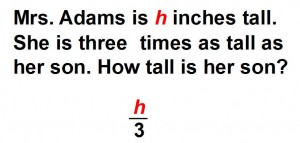# Verbal expressions in algebra

Verbal expressions in mathematics are standardized terms that help alleviate confusion created by the attempts to explain mathematical expressions using words. Each element and operator in a mathematical equation has its own name and there are standardized phrases that are used to describe the relationships between them. Putting it plainly, they help you turn sentences like: “The second number on the left, behind the little cross, has a small number 2 a bit to the right and above it and then there is the small letter x and then 17 and after that two, short lines on top of one another then number 4” into something people can actually understand. Using examples, we are going to list the verbal expressions you are going to need to communicate all the basic mathematical expressions you want.## Verbal expressions in algebra exams for teachers

 Exam Name File Size Downloads Upload date Express algebraically Without variables Verbal expressions algebraically – No variables – sum 10.0 kB 5456 December 5, 2011 Verbal expressions algebraically – No variables – difference 0 B 2671 January 1, 1970 Verbal expressions algebraically – No variables – product 0 B 2225 January 1, 1970 Verbal expressions algebraically – No variables – quotient 0 B 3003 January 1, 1970 Verbal expressions algebraically – No variables – exponenting 0 B 2160 January 1, 1970 Into equations Verbal expressions algebraically – Equation – sum 0 B 4922 January 1, 1970 Verbal expressions algebraically – Equation – difference 0 B 2958 January 1, 1970 Verbal expressions algebraically – Equation – product 7.9 kB 2587 December 5, 2011 Verbal expressions algebraically – Equation – quotient 0 B 2837 January 1, 1970 Verbal expressions algebraically – Equation – exponenting 0 B 2148 January 1, 1970 Into expression Verbal expressions algebraically – Expression – sum 8.0 kB 3745 December 5, 2011 Verbal expressions algebraically – Expression – difference 0 B 2529 January 1, 1970 Verbal expressions algebraically – Expression – product 0 B 2553 January 1, 1970 Verbal expressions algebraically – Expression – quotient 0 B 5255 January 1, 1970 Verbal expressions algebraically – Expression – exponenting 0 B 2068 January 1, 1970 Into inequalities Verbal expressions algebraically – Inequality – sum 0 B 6756 January 1, 1970 Verbal expressions algebraically – Inequality – difference 0 B 1854 January 1, 1970 Verbal expressions algebraically – Inequality – product 0 B 3726 January 1, 1970 Verbal expressions algebraically – Inequality – quotient 0 B 6952 January 1, 1970 Verbal expressions algebraically – Inequality – exponenting 0 B 1525 January 1, 1970 Express with words Without variables Algebraic expressions with words – No variables – sum 7.3 kB 2191 December 5, 2011 Algebraic expressions with words – No variables – difference 0 B 1371 January 1, 1970 Algebraic expressions with words – No variables – product 0 B 1359 January 1, 1970 Algebraic expressions with words – No variables – quotient 0 B 1922 January 1, 1970 Algebraic expressions with words – No variables – exponenting 0 B 1300 January 1, 1970 Into equations Algebraic expressions with words – Equation – sum 0 B 2142 January 1, 1970 Algebraic expressions with words – Equation – difference 0 B 2999 January 1, 1970 Algebraic expressions with words – Equation – product 0 B 1543 January 1, 1970 Algebraic expressions with words – Equation – quotient 0 B 2001 January 1, 1970 Algebraic expressions with words – Equation – exponenting 0 B 1580 January 1, 1970 Into expressions Algebraic expressions with words – Expression – sum 0 B 1919 January 1, 1970 Algebraic expressions with words – Expression – difference 0 B 1382 January 1, 1970 Algebraic expressions with words – Expression – product 0 B 1511 January 1, 1970 Algebraic expressions with words – Expression – quotient 0 B 1503 January 1, 1970 Algebraic expressions with words – Expression – exponenting 0 B 1467 January 1, 1970 Into inequalities Algebraic expressions with words – Inequality – sum 0 B 2246 January 1, 1970 Algebraic expressions with words – Inequality – difference 0 B 1573 January 1, 1970 Algebraic expressions with words – Inequality – product 0 B 1821 January 1, 1970 Algebraic expressions with words – Inequality – quotient 0 B 2847 January 1, 1970 Algebraic expressions with words – Inequality – exponenting 0 B 1281 January 1, 1970 Evaluate expression of number Evaluate verbal expressions of number – sum 0 B 2183 January 1, 1970 Evaluate verbal expressions of number – difference 0 B 1449 January 1, 1970 Evaluate verbal expressions of number – product 0 B 1509 January 1, 1970 Evaluate verbal expressions of number – quotient 0 B 2252 January 1, 1970 Evaluate verbal expressions of number – exponenting 0 B 2222 January 1, 1970

## Verbal expressions in algebra worksheets for students

 Worksheet Name File Size Downloads Upload date Verbal expressions algebraically Verbal expressions algebraically – No variables 0 B 2492 January 1, 1970 Verbal expressions algebraically – Equations 0 B 25081 January 1, 1970 Verbal expressions algebraically – Expressions 0 B 3427 January 1, 1970 Verbal expressions algebraically – Inequalities 0 B 25081 January 1, 1970 Algebraic expressions with words Algebraic expressions with words – No variables 0 B 1611 January 1, 1970 Algebraic expressions with words – Equations 0 B 2528 January 1, 1970 Algebraic expressions with words – Expressions 0 B 2313 January 1, 1970 Algebraic expressions with words – Inequalities 0 B 1527 January 1, 1970 Evaluate verbal expressions of number Evaluate verbal expressions of number 0 B 3019 January 1, 1970